`Learn Biostatistics with Ria`

Come on this incredible journey and help enhance your capability for Biomedical Research

# INDEPENDENT T-TEST

The previous blogs on Chi-square and Fisher’s Exact Test deal with Categorical data (frequency) and are not-normal in distribution. Hence the tests are non-parametric in nature. However, the data which ARE normal, can be represented like this:

# Normal Distribution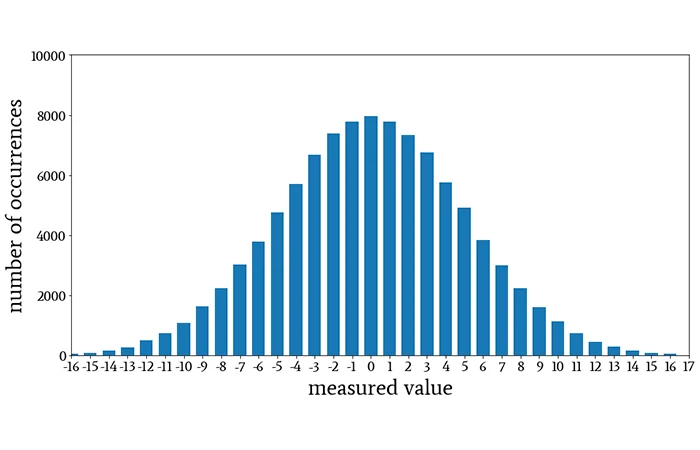This is called continuous data i.e. the data which can be measured on a scale, with a real zero (0) being also a value somewhere in there, and sometimes an important one at that.

If we need a point estimate from any normally distributed dataset, the one we use is Mean. It is the arithmetic mean of all the values in the dataset.

#### Mean (x̄) = Ʃ xi / n

Where denotes the mean statistic of the sample, i is the individual value, and n is the sample size. But what about the rest of the data in the dataset? Can we just disregard them? When we were children, we used to, for simplification. But turns out we cannot anymore since the Mean alone cannot paint the whole picture of how the dataset is. Consider the following:

# Same Mean with different Variability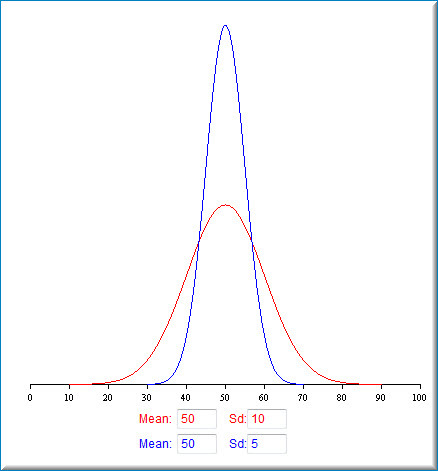These two datasets represented here, even though with the same Mean value, are completely different in nature. That is because of the other characteristic seen here: SD, or the Standard Deviation. It is the representation of the dispersion of the data points around the Mean value. It is a simple enough process to calculate Standard Deviation. Imagine all these data points:

 Participant number Quiz score out of 20 (xi) Deviation from the Mean (xi - x̄) (xi - x̄)2 1 10 10 – 12.3 = -2.3 5.29 2 15 15 – 12.3 = 2.7 7.29 3 17 17 – 12.3 = 4.7 22.09 4 13 13 – 12.3 = 0.7 0.49 5 8 8 – 12.3 = -4.3 18.49 6 11 11 – 12.3 = -1.3 1.69 MEAN 74/6 = 12.3 TOTAL SQUARED DEVIATION 55.34

The deviations from the mean were all squared to bring all of them on the same sign, otherwise, they would have negated each other out while calculating the total. Thus the total squared deviation (55.34) when divided by the number of observations, gives the average of the squared deviations, or Variance.

In the sample calculation for Variance, since this is a limited dataset, we put (n-1) instead of n. The rationale behind this is, that if we know the values for (n-1) data, we can obviously figure out the nth value, creating a bias. The calculations for both the sample standard deviation and the sample variance both contain a little bias or “error”. Bessel's correction (i.e. subtracting 1 from your sample size) corrects this bias.

Variance (s2) = Ʃ (xi - x̄)2 / (n-1)

But this is a squared value. When we dial it back by doing the square root, we get the Standard Deviation of the dataset.

# Standard Deviation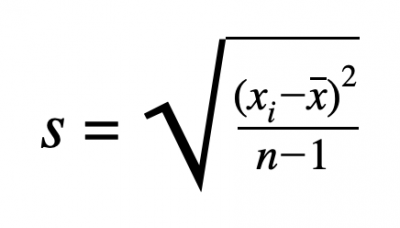Note: When in population, we no longer use the sample statistic like x̄ or s. We use population parameters like μ (pronounced mu = population mean) and σ (pronounced sigma = population standard deviation).

# Sample & Population Standard Deviation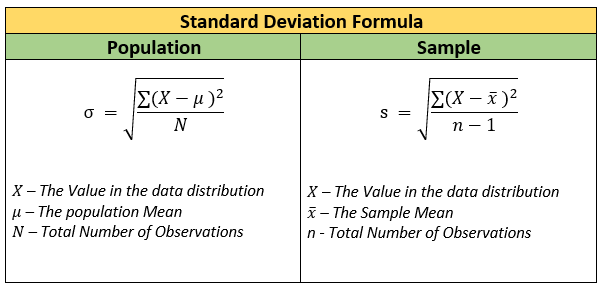In our example: the standard deviation is s = √(55.34/5) = 3.35

We can interpret these values as:

The mean score of the 6 participants is 12.3 ± 3.35. This means that 68% of the data points lie within ± 3.35 score or 1 standard deviation from the mean score. Similarly, 95% of the data points lie within 2 standard deviations, and 99.7% lie within 3 standard deviations of the mean score.

# Distribution of data points around Mean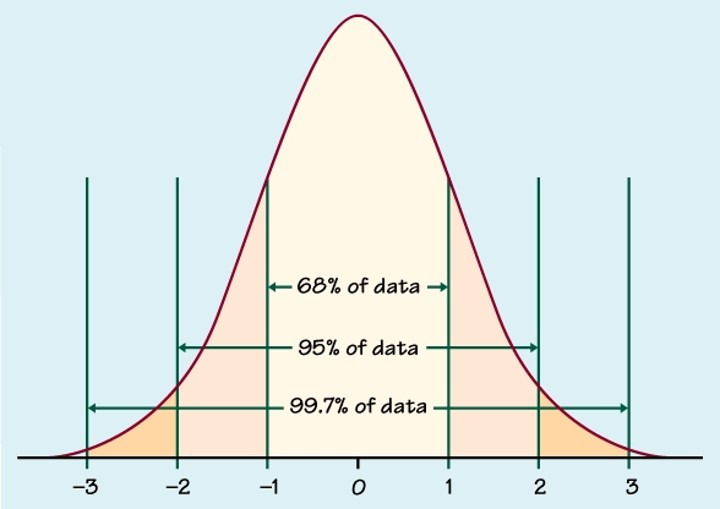# NORMALITY

There are many different methods to know whether the dataset is distributed normally or not:

1) You can calculate the Mean, Median, and Mode from the tabular representation of this data. If all three are equal (Mean = Median = Mode), the data shows the normal distribution.

2) If the Mean of the dataset is 0 with the variance 1, then the data is normally distributed (in a sample size of at least 50, if the Standard deviation is less than half of the mean, then the data is said to be normally distributed).

3) The values of Skewness and Kurtosis. Either an absolute skewness value between ‐2 to +2 or an absolute kurtosis (excess) value ≤ 4 are used as reference values for determining considerable normality, as said by Kim HY (2013).

4) The Shapiro-Wilk Test or the Kolmogorov-Smirnov Test (if the p-value is more than 0.05, then the data is normally distributed).

5) Graphical representation by Histogram and Frequency Polygon (if the graph is approximately bell-shaped or symmetric around the mean, normality is present).

6) Graphical representation by P-P plot or Q-Q plot (forms an approximately straight line).

7) Graphical representation by Box-Whisker plot (median represents the horizontal line inside the box, Interquartile Range the length of the box, the whiskers are the minimum and maximum values 1.5 times the IQR from either end of the box). A box plot that has the median at the center of the box with symmetric whiskers indicates normality.

# INDEPENDENT T-TEST

Now that we are clear on the basics of normality, let us move on to the Independent t-test. See the following examples:

1. A drug company may want to test a new cancer drug to find out if it improves life expectancy. In an experiment, there’s always a control group who are given a placebo. The control group may show an average life expectancy of +5 years, while the group taking the new drug might have a life expectancy of +6 years. It seems that the drug might work. But it could be due to a fluke too. How to know whether there is actually a difference in outcome?

2. A researcher decided to investigate whether an exercise or weight loss intervention is more effective in lowering cholesterol levels. To this end, the researcher recruited a random sample of inactive males that were classified as overweight. This sample was then randomly split into two groups: Group 1 underwent a calorie-controlled diet and Group 2 undertook the exercise-training program. In order to determine which treatment program was more effective, the mean cholesterol concentrations were compared between the two groups at the end of the treatment programs.

3. Some island has 1,000 male and 1,000 female inhabitants. An investigator wants to know if males spend more or fewer minutes on the phone each month. Ideally, he'd ask all 2,000 inhabitants but this takes too much time. So he samples 10 males and 10 females and asks them. These sample means differ by some (99 - 106 =) -7 minutes: on average, females spend some 7 minutes less on the phone than males.

Essentially in each of these examples, we want to find out if the difference in mean values of the two groups in the sample reflect a true difference in the population or not.

Usually to compare differences between samples with known population variance (σ2), we use Z test. The formula is:

# Z test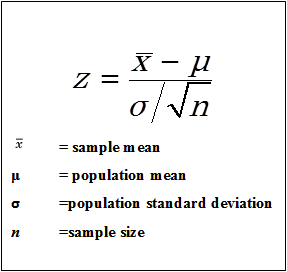But it is not always possible to know the population variance, and we have to calculate an estimate of the same from the sample variances of the two groups. It is only then we use the Independent t-test. Using an independent t-test is appropriate only if your data "passes" 6 assumptions that are required for an independent t-test to give you a valid result.

1. The dependent variable should be continuous in nature (it is measured at the interval or ratio level).

2. The independent variable should consist of two categorical, independent groups.

3. There should be independence of observations, which means that there is no relationship between the observations in each group or between the groups themselves. For example, there must be different participants in each group with no participant being in more than one group.

4. There should be no significant outliers. Outliers are simply single observations within the data that do not follow the usual pattern, such as values that are >1.5 times the IQR in a box-whisker plot of the dataset. The problem with outliers is that they can have a negative effect on the independent t-test, reducing the validity of the results (e.g. in a study of 100 students' IQ scores, where the mean score was 108 with only a small variation between students, one student had a score of 156, which is very unusual, and may even put her in the top 1% of IQ scores globally).

5. The dependent variable should be approximately normally distributed for each group of the independent variable. We talk about the independent t-test only requiring approximately normal data because it is quite "robust" to violations of normality, meaning that this assumption can be a little violated and still provide valid results.

6. There needs to be homogeneity of variances among the groups of independent variables.

A. Null Hypothesis (H0) : There is no significant difference between the mean values of the two groups, i.e. μ1 = μ2 or μ1 μ2 = 0

B. Alternate Hypothesis (Ha) : There is a significant difference between the mean values of the two groups, i.e. μ1 ≠ μ2 or μ1 μ2 ≠ 0

C. Now each group will have a mean value and a variance. Suppose:

Group 1 (size n1): Mean x̄1 and variance s12

Group 2 (size n2): Mean x̄2 and variance s22

The pooled variance (the weighted average of the variance estimates from the two groups) can be calculated as follows:

· If n1 = n2 then the formula simplifies. When the group sizes are equal, the pooled variance reduces to sp2 = ( s12+ s22)/2, which is the average of the two variances.

· However, if n1 ≠ n2 then to find the weighted average, we have to factor in the group sizes. The formula is sp2 = Σ(ni−1)si2/Σ(ni−1) which roughly translates to:

# Pooled variance from 2 groups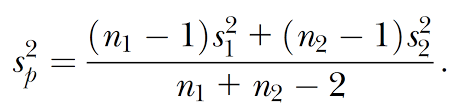# D. The value of the t-test can be calculated as: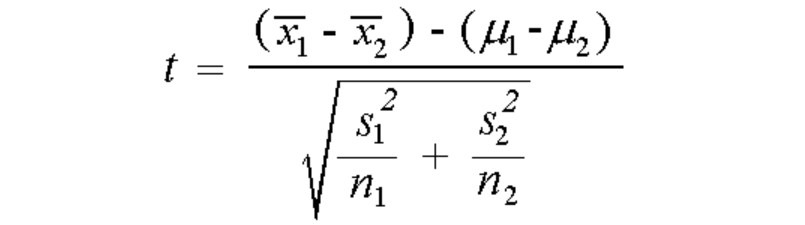# If we use the pooled standard deviation, we can also write like: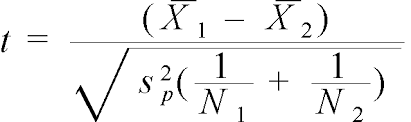E. The degree of freedom for this t-test is (n1+n2-2). At a certain level of significance, such as 5%, there is a critical value of the t-test at (n1+n2-2) degrees of freedom.

If the calculated value is greater than the critical or tabulated value (tcal > ttab), we can reject the Null hypothesis and say that the value of the t-test shows a significant difference in the mean values of the two groups (Statistical decision).

# t-test table for critical values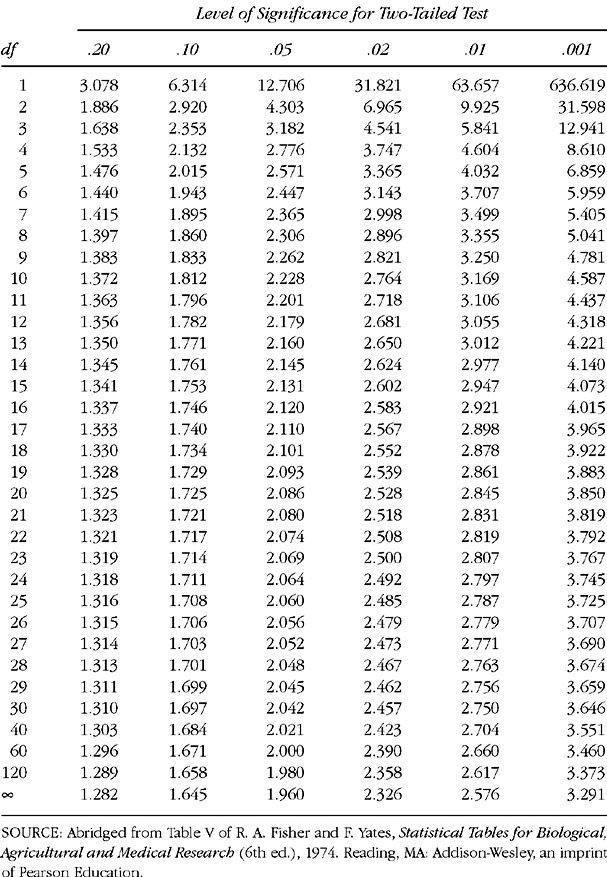F. For this significant test, we can conclude that p <0.05.

Let us now practice:

# Example

The purpose of the study by Ingle and Eastell was to examine the bone mineral density (BMD) and ultrasound properties of women with ankle fractures. The investigators recruited 31 postmenopausal women with ankle fractures and 31 healthy postmenopausal women to serve as controls. One of the baseline measurements was the stiffness index of the lunar Achilles. The mean stiffness index for the ankle fracture group was 76.9 with a standard deviation of 12.6. In the control group, the mean was 90.9 with a standard deviation of 12.5. Do these data provide sufficient evidence to allow you to conclude that, in general, the mean stiffness index is higher in healthy postmenopausal women than in postmenopausal women with ankle fractures? Let α = 0.05.

H0: The mean stiffness index is equal in both groups of postmenopausal women.

Ha: The mean stiffness index is not equal in the two groups of postmenopausal women.

Healthy postmenopausal women group: x̄1 = 90.9 and s1 = 12.5

Postmenopausal women group with ankle fracture: x̄2 = 76.9 and s2 = 12.6

Since n1 = n2 , the pooled standard deviation is the average of the two standard deviations i.e. sp = √[( s12+ s22)/2] = √[(12.52 + 12.62)/2] = 12.55

The value of t-test at α = 0.05, and (31+31-2) or 60 degrees of freedom, is:

t = (x̄1 - 2)/[ sp √(1/ n1 + 1/ n2)] = (90.9-76.9)/[12.55 √(1/31 + 1/31)]

= 14.0 / 3.18 = 4.4

The critical value of t at α = 0.05, and 60 degrees of freedom is 2 (see the previous table).

Since tcal > ttab we can reject the Null hypothesis and declare that there is a statistically significant difference in the mean stiffness index between the two groups. Since the mean stiffness index is higher in healthy postmenopausal women in the sample, we can conclude:

In the general population, the mean stiffness index is significantly higher in healthy postmenopausal women than in postmenopausal women with ankle fractures (p<0.05).

Do by yourself:

A test designed to measure mothers’ attitudes toward their labor and delivery experiences was given to two groups of new mothers. Sample 1 (attenders) had attended prenatal classes held at the local health department. Sample 2 (nonattenders) did not attend the classes. The sample sizes and means and standard deviations of the test scores were as follows:

 Sample n x̄ s 1 15 4.75 1.0 2 22 3.00 1.5

Do these data provide sufficient evidence to indicate that attenders, on average, score higher than nonattenders? Let α = 0.05.

BIBLIOGRAPHY:

1. Mishra P, Pandey CM, Singh U, Gupta A, Sahu C, Keshri A. Descriptive statistics and normality tests for statistical data. Ann Card Anaesth 2019;22:67-72.

2. Wayne W. Daniel, Chad L. Cross. Biostatistics A Foundation for Analysis in the Health Sciences. Wiley Publishers, United States. 2013; 10: 24-237.

# NEXT UP

Mann Whitney U test

Written by:

Dr. Ria Roy
Senior Resident
Department of Community and Family Medicine, AIIMS Patna

Interests: Adolescent Health, Nutrition, Biostatistics, Epidemiology, NCDsLaunch your Graphy
100K+ creators trust Graphy to teach online